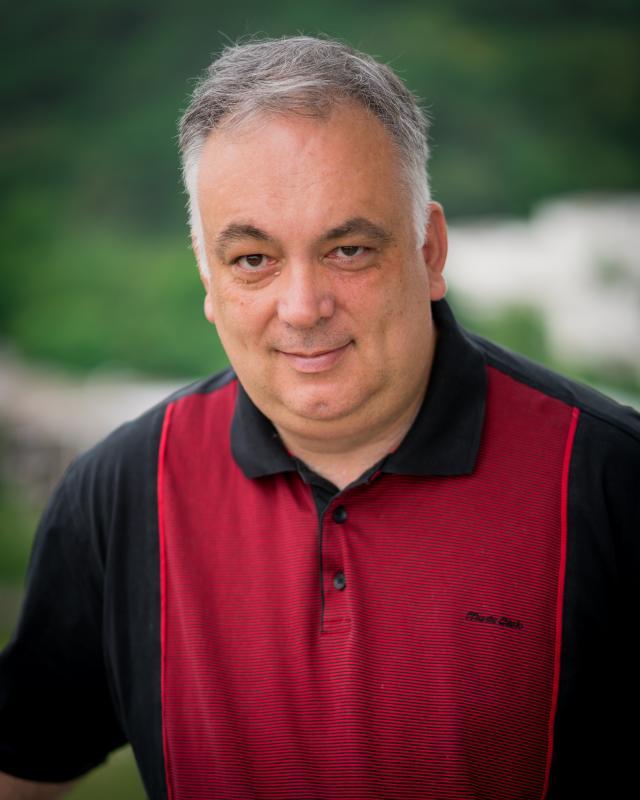Department of Mathematics

# Areas of Research

The six institutes in the department cover many important research areas in mathematics.

The Department of Mathematics conducts research in many important and modern fields of mathematics. On the one hand, strong fundamental research and the interplay between mathematical fields play a key role. On the other hand, research topics are inspired by interdisciplinary questions in natural, engineering, life and economic sciences, leading to the development of new mathematical methods and foundations.

Members of the Department of Mathematics participate in the Cluster of Excellence SimTech and in various Collaborative Reseach Centers and Research Training Groups. They maintain strong international and national collaborations, and sustain several large software projects.

## Research Areas

 Prof. Dr. M. Geck Algebra, Representation theory, algebraic groups and Lie-Algebren, Computer algebra Prof. Dr. A. Henke Representation theory of groups and algebras, Combinatorial representation theory, Algebraic combinatorics Prof. Dr. S. König Algebra, Representation theory, Homological and categorical structures
 Prof. Dr. M. Griesemer Dynamics and spectral theory of many-particle quantum systems, Variational methods in quantum mechanics Prof. TeknD T. Weidl Spectral theory, Mathematical physics Apl. Prof. Dr.  J. Wirth Analysis of partial differential equations, Operator theory, Harmonic analysis in Rn and on Lie groups Prof. Dr. F. Witt global Analysis

 Apl.Prof. Dr. W.-P. Düll Nonlinear partial differential equations, Mathematical fluid mechanics, Justification of approximation equations, Multi-scale methods in mathematical modelling Prof. Dr. J. Pöschel Dynamical systems, Stability of Hamiltonian systems, KAM-theory Prof. Dr. C. Scherer Systems theory and optimisation, Analysis of uncertain dynamical systems, Robust controller synthesis Prof. Dr. G. Schneider Nonlinear partial differential equations, Diffusive and dispersive dynamics, Multi-scale problems in mathematical modelling

 Priv.-Doz. Dr. A. Degeratu Differential geometry, Complex geometry, Partial differential equations, Gauge theory, Mathematical physics, Mathematical biology Prof. Dr. M. Eisermann Geometric topology, Low-dimensional manifolds, Group theory, Algorithms and computer algebra Priv.-Doz. Dr. M. Hamilton 4-dimensional manifolds, Geometric topology, Mathematical and theoretical physics Apl. Prof.  Dr. A. Kollross Lie group actions, Riemannian homogeneous and symmetric spaces Prof. Dr. U. Semmelmann Spin geometry and Dirac operators, Geometric differential equations, Holonomy theory and special geometric structures Prof. Dr. F. Witt Differential and algebraic geometry

 Priv.-Doz. Dr. A. Degeratu Differential geometry, Complex geometry, Partial differential equations, Gauge theory, Mathematical physics, Mathematical biology Apl.Prof. Dr. W.-P. Düll Nonlinear partial differential equations, Mathematical fluid mechanics, Justification of approximation equations, Multi-scale methods in mathematical modelling Prof. Dr. M. Griesemer Dynamics and spectral theory of many-particle quantum systems, Variational methods in quantum mechanics Priv.-Doz. Dr. P. Lesky Partial differential equations, Spectral theory for self-adjoint operators, Energy estimates for solutions of nonlinear wave equations Prof. Dr. J. Pöschel Dynamical systems, Mathematical and theoretical physics, KAM-theory Prof. TeknD T. Weidl Spectral theory, Mathematical physics Prof. Dr. F. Witt Quantum Theory

 Apl. Prof. Dr. W.-P. Düll Nonlinear partial differential equations, Mathematical fluid mechanics, Justification of approximation equations, Multi-scale methods in mathematical modeling Prof. Dr. B. Haasdonk Model reduction and data-based modelling, Numerical simulation, Machine learning Priv.-Doz. Dr. I. Rybak Flows in porous media, numerical methods for multi-scale problems Prof. Dr. G. Schneider Nonlinear partial differential equations, Diffusive and dispersive dynamics, Multi-scale problems in mathematical modelling

 Prof. Dr. A. Barth Stochastic partial differential equations, Uncertainty quantification, Stochastic analysis Prof. Dr. D. Göddeke High performance computing, GPU computing, Applications in optimisation, geo- and life sciences Prof. Dr. B. Haasdonk Model reduction and data-based modelling, Numerical simulation, Machine learning Prof. Dr. C. Rohde Modelling, analysis, simulation with nonlinear partial differential equations, Mathematical fluid mechanics / CFD Priv.-Doz. Dr. I. Rybak Flows in porous media, Numerical methods for multi-scale problems
 Prof. Dr. C. Scherer Systems theory and optimisation, Analysis of uncertain dynamical systems, Robust controller synthesis

 Priv.-Doz. Dr. A. Degeratu Differential geometry, Complex geometry, Partial differential equations, Gauge theory, Mathematical physics, Mathematical biology Apl. Prof. Dr. W.-P. Düll Nionlinear partial differential equations, Mathematical fluid mechanics, Justification of approximation equations, Multi-scale methods in mathematical modelling Prof. Dr. C. Rohde Modelling, analysis, numerics with nonlinear partial differential equations, Mathematical fluid mechanics / CFD Prof. Dr. G. Schneider Nonlinear partial differential equations, Diffusive and dispersive dynamics, Multi-scale problems in mathematical modelling Apl. Prof. Dr.  J. Wirth Analysis of partial differential equations, Operator theory, Harmonic analysis in Rn and on Lie groups

 Prof. Dr. A. Barth Stochastic partial differential equations, Uncertainty quantification, Stochastic analysis Priv.-Doz. Dr. J. Dippon Stochastic analysis, Stochastic models in biology and medicine, Mathematical finance Prof. Dr. C. Hesse Nonparametric theory of estimation, especially deconvolution, Statistical analysis of voting systems, Differential equations with random initial conditions Prof. Dr. I. Steinwart Statistical learning theory and machine learning, Cluster analysis, neural networks
 Prof. Dr. M. Eisermann Geometric topology, in particular low-dimensional manifolds, Group theory, Algorithms and computer algebra

 Prof. Dr. D. Göddeke High performance computing, GPU computing, Applications in optimisation, geo- and life sciences Prof. Dr. I. Steinwart Efficient implementations of learning algorithms, Applications for learning algorithms, neural networks

### Contact person for specific project inquiries:Prof. TeknD